﻿ Table 1. The Main differences between Molecularity and Order of Reaction

# Table 1. The Main differences between Molecularity and Order of Reaction

 Moleculariy of Reaction Order of Reaction It is the total number of reacting species (molecules, atoms or ions) which bring the chemical change. It is the sum of powers of molar concentration of the reacting species in the rate equation of the reaction. It is always a whole number. It may be a whole number, zero, fractional. It is a theoretical concept. It is experimentally determined. It is meaningful only for simple reactions or individual steps of a complex reaction. It is meaningless for overall complex reaction. It is meant for the reaction and not for its individual steps

Factors Affecting Rate of Reaction:

1) Nature of reactants:

a) Physical state of reactants: This has considerable effect over rate of reaction: Gaseous state > Liquid state > Solid state —> Decreasing rate of reaction

b) Physical size of the reactants: Among the solids, rate increases with decrease in particle size of the solid.

c) Chemical nature of the reactants:

· Reactions involving polar and ionic substances including the proton transfer reactions are usually very fast. On the other hand, the reaction in which bonds is rearranged, or electrons transferred are slow.

· Oxidation-reduction reactions, which involve transfer of electrons, are also slow as compared to the ionic substance.

· Substitution reactions are relatively much slower.

2) Effect of temperature: The rate of chemical reaction generally increases on increasing the temperature. The rate of a reaction becomes almost double or tripled for every rise in temperature.

Temperature coefficient (j) of a reaction is defined as the ratio of rate constants at two temperatures differing by 10°C: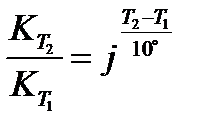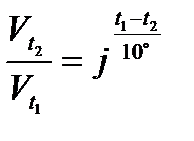or

Arrhenius proposed a quantitative relationship between rate constant and temperature as,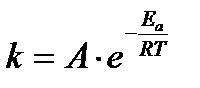The equation is called Arrhenius equation. In which constant A is known as frequency factor. This factor is related to number of binary molecular collision per second per litre; Ea is the activation energy; T is the absolute temperature and R is the gas constant. Both A and Ea are collectively known as Arrhenius parameters.

Taking logarithm equation may be written as, log k = log A – Ea/2.303 RT

The value of activation energy (Ea) increases, the value of k decreases and therefore, the reaction rate decreases.

The physical meaning of the activation energy (Ea) is that it is the minimum relative kinetic energy which the reactant molecules must possess for changing into the products molecules during their collision. This means that the fraction of successful collision is equal to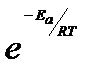called Boltzmann factor.

The Arrhenius equation can be written in a non-exponential form, which is often more convenient to use and to interpret graphically. Taking the natural logarithms of both sides and separating the exponential and pre-exponential terms yields: ln(k) = ln(A)−EaRT

Note that this equation is of the form y = mx + b, and creating a plot of ln(k) versus 1/T will produce a straight line with the slope –Ea/R.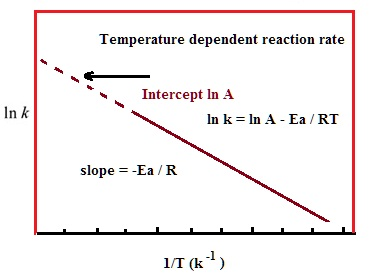This affords a simple way of determining the activation energy from values of k observed at different temperatures. We can plot ln(k) versus 1/T,and simply determine the slope to solve for Ea.

The activation energy can also be calculated algebraically if k is known at two different temperatures:

At temperature 1: ln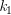= –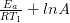At temperature 2: ln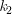= –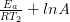We can subtract one of these equations from the other: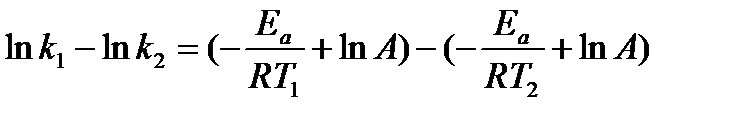This equation can then be further simplified to: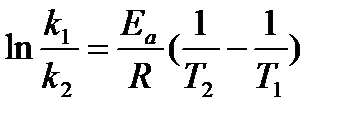or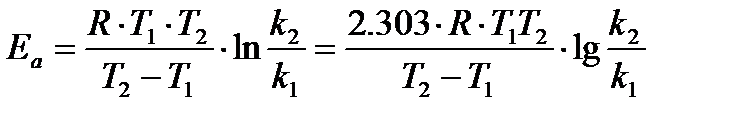3) Concentration of reactants: In 1864,Peter Waage and Cato Guldberg pioneered the development of chemical kinetics by formulating the law of mass action, which states that the speed of a chemical reaction is proportional to the quantity of the reacting substances.

The rate at which a substance reacts is directly proportional to its active mass and the rate at which a reaction proceeds is proportional to the product of the active masses of the reacting substances: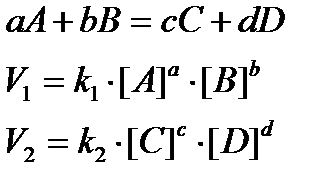• For a reaction, aA + bB –> product

Rate = (dx/dt) ∝ [A]a[B]b; (dx/dt) = k[A]a[B]b

where k is called rate constant or velocity constant.

When [A] = [B] = 1 mol/litre, then dx/dt = k

Thus, rate constant k is also called specific reaction rate.

• The value of rate constant depends on, nature of reactant, temperature and catalyst. It is independent of concentration of the reactants.

• Unit of rate constant = [litre/mol]n–1 × sec–1 = [mol/litre]1–n × sec–1

Where n order of reaction.

4) Presence of catalyst: a catalyst is a substance, which increases the rate of a reaction without itself being consumed at the end of the reaction, and the phenomenon is called catalysis. here are some catalysts which decrease the rate of reaction and such catalysts are called negative catalyst. Obviously, the catalyst accelerating the rate will be positive catalyst. However, the term positive is seldom used and catalyst itself implies positive catalyst.

Catalyst are generally foreign substances but sometimes one of the product formed may act as a catalyst and such catalyst is called “auto catalyst” and the phenomenon is called auto catalysis.

The function of a catalyst is to lower down the activation energy. The greater the decrease in the activation energy caused by the catalyst, higher will be the reaction rate.

5) Effect of sunlight: There are many chemical reactions whose rates are influenced by radiations particularly by ultraviolet and visible light. Such reactions are called photochemical reactions. For example, Photosynthesis, Photography, Blue printing, Photochemical synthesis of compounds etc.

The radiant energy initiates the chemical reaction by supplying the necessary activation energy required for the reaction.

Дата добавления: 2017-05-02; просмотров: 1482; ЗАКАЗАТЬ НАПИСАНИЕ РАБОТЫ

Поиск по сайту:

Воспользовавшись поиском можно найти нужную информацию на сайте.

Поделитесь с друзьями:

Считаете данную информацию полезной, тогда расскажите друзьям в соц. сетях.
﻿Poznayka.org - Познайка.Орг - 2016-2023 год. Материал предоставляется для ознакомительных и учебных целей.
Генерация страницы за: 0.027 сек.